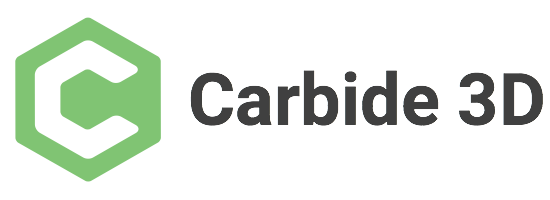# Upgrading a Sparkfun SO3 to GRBL 1.1

(Reiner) #1

Today I finally found the courage to try the GRBL 1.1 / CarbideMotion 4.0 upgrade on my Shapeoko 3 Sparkfun Edition. Since I faced some issues (and was able to solve them as far as I can see) I thought I share my experience here.

Since I had already installed a set of 5 limit switches (Z, +X, -X, +Y, -Y), so I thought I would try the Carbide Upgrader tool from the Carbide3D website. I started the tool and could connect to the machine after selecting the Arduino device - the Stepoko board of the SO3 Sparkfun Edition does not appear as a Carbide3D device like the original board.

I then pressed the Z limit switch and the program was able to flash correct but later failed during the step “Checking success.” where it presented the device selection window again, but was apparently not able to correctly connect to check the success. Since the previous step “Programming.” was successful, I decided to risk it and cancel the Upgrader here.

I then started CarbideMotion 4.0 and was able to connect to the SO3 but during the homing process, the spindle did only move down on the Z axis about 5mm and then everything stopped in error. Sometimes I got the “Door open” error that other people here have seen before.

I was stuck at that point and decided that I would try with Universal G-code Sender (UGS) first, as I get a little bit more information there. In UGS I could see that the movements did not work as expected and I started to check my GRBL settings with the \$\$ command. I had to set \$3=5 (reverse direction on X and Z axis) and \$5=1 (limit switches inverted) as my limit switches are of the normally closed type and wired in series on the X and Y axis. After that I was able to home the machine with \$H in UGS.

I then tried again with CarbideMotion 4.0 and now homing and jogging around also worked there. I haven’t had the time yet to do much more testing, but beeing able to home, move and rapid position the machine in CarbideMotion seems promising.

I am still a little unsure if I need to set more settings in GRBL. My current settings are:

``````\$0=10
\$1=255
\$2=0
\$3=5
\$4=0
\$5=1
\$6=0
\$10=255
\$11=0.020
\$12=0.010
\$13=0
\$20=0
\$21=0
\$22=1
\$23=0
\$24=100.000
\$25=1000.000
\$26=25
\$27=5.000
\$30=1000
\$31=0
\$32=0
\$100=40.000
\$101=40.000
\$102=40.000
\$110=5000.000
\$111=5000.000
\$112=5000.000
\$120=400.000
\$121=400.000
\$122=400.000
\$130=425.000
\$131=465.000
\$132=80.000
``````

If somebody with a “real” SO3 could have a look at these settings and compare them and tell me what is set there, that would be great.

Reiner

3 Likes

GRBL 1.1 on Red Shapeoko Let the Fun Begin
(fredmud) #2

Replying here so that other searching Sparkfun SO3 owners might have an easier time.

The Carbide Upgrader does not work, as it will not recognize the Stepoko (Sparkfun Arduino board).

I found great, detailed instructions to updating GRBL via the Arduino IDE at:

I then went in with UGS to reconfigure my GRBL settings (probably also doable using Carbide Motion). I have limit switches, and my settings reflect that:

\$0=30 (Carbide’s step pulse default setting is 10, but I’ve kept mine at Sparkfun’s default 30. Haven’t experimented with that.)
\$1=255
\$2=0
\$3=0
\$4=0
\$5=1
\$6=0
\$10=155 (Carbide’s default is 255. This config has apparently changed in 1.1, though, and only recognizes 0,1, or 2?)
\$11=0.020
\$12=0.010
\$13=0
\$20=0
\$21=1
\$22=1
\$23=0
\$24=25.000 (conservative)
\$25=1000.000
\$26=25
\$27=1.000 (maximizing usable area; Carbide’s homing pull-off default is 5.000)
\$30=1000 (spindle, does not apply)
\$31=0 (spindle, does not apply)
\$32=0 (laser, does not apply)
\$100=39.936 (default is 40; this is my unique machine calibration)
\$101=39.936 (ditto)
\$102=40.733 (ditto)
\$110=5000.000
\$111=5000.000
\$112=5000.000
\$120=400.000
\$121=400.000
\$122=400.000
\$130=415.000 (Carbide’s default is 425, but with limit switches, I don’t have that much travel)
\$131=435.000 (ditto; default 465)
\$132=80.000

I hope this info helps you, Reiner, if you are still having problems, and anyone else who is updating their now unsupported by Sparkfun machine.

0 Likes

(andrew pargeter) #3

Thanks for putting up al this useful information. I wanted to do the same thing but was afraid to mess up the board. Carbide sold me their board. It was expensive and came WITHOUT a power supply and heatsink. I’ll have to 3D print an enclosure as well. I might just send it back if I can do what you have done. Can you provide the compiled file so I can flash the Stepeoko?

Thanks much,
Andrew

0 Likes

(Reiner) #4

I used this binary file: http://carbide3d.com/dl/v4/grbl.hex
and the Updater from https://docs.carbide3d.com/support/carbideupdater/

1 Like